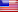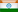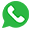(+1) 408-400-3668(+91) 913-696-0293# Pattern Program In Python

By Tech-Act
08/17/2023  3283 ViewsCracking interviews is a tough task and especially when the interview is about a programming language like Python then you shouldn’t take any chance and must be well prepared. If you are going for a Python interview then the chances are very high that they might test your knowledge about Pattern programs in Python. Hence, it is extremely important for you to understand Pattern program in Python. Learn Python step-by-step.

So today, in this article we will elaborate on Python pattern programs. I would recommend you to be not in hurry to just run through the article rather read it very carefully so that you understand what part of code does what before you move on to the next pattern. If you don’t understand them in depth than your concept will not be clear hence you would fail to explain the same to the interviewer. So, without wasting any further time let’s get started.

#### We are beginning with the basic patterns

• Number Pattern Problem
• Incrementing Number Pattern Problem
• Pyramid Pattern Problem with Stars
• Inverted Semi-Pyramid Pattern Problem with Numbers

Let’s Begin….

#### 1. Number Pattern Problem

The pattern we want to form should look like as following:

1

2 2

3 3 3

4 4 4 4

5 5 5 5 5

Let’s go ahead and see how can we print this number pattern programs in python:

depth = 6

for number in range(depth):

for i in range(number):

print(number, end=” “)

print(” “)

Code Interpretation:

We start off by initializing a variable called “depth” and give it a value of 6 with the help of this command:

depth = 6

Going ahead, we have a nested for loop. We have the outer for loop to set the depth or number of rows in this pattern. When we use the command:

for number in range(depth):

This helps us to get a list of numbers 0-5. Because the depth value is 6, this means that 6 is exclusive and that is why the range goes from 0-5. Then, we set the inner for loop using this command:

for i in range(number).

In the inner for loop, initially, the value of the number is 0, that is why we skip the first row. Then we go back to the outer for loop and the number value becomes 1. Once the number value is 1, we head inside the inner loop and print 1. After that number value is incremented again and it becomes 2, then we head to the inner loop and print 2 2. Similarly, we go back to the outer loop and this time number value becomes 3. After this inside the for loop, we print 3 3 3. Further which, again we go back to the outer loop, then the value of “number “becomes 4 and we print 4 4 4 4 with the inner loop. Finally, we will print 5 5 5 5 5.

#### 2. Incrementing Number Pattern Problem

The pattern we want to form should look like as following

1

1 2

1 2 3

1 2 3 4

1 2 3 4 5

Now, let’s go ahead and see how we can have a sequence of numbers in each row with python:

depth = 6

for number in range(1, depth):

for i in range(1, number + 1):

print(i, end=’ ‘)

print(“”)

Code Interpretation:

We start off by initializing the value of depth to be 6. Then, we set the outer for loop with this command:

for number in range(1, depth)

With the help of this command, we create a range of numbers which go on from 1 to 5. Then, we go ahead and set the inner for loop with this command:

for i in range(1, number + 1).

Inside the for loop, we print the digits using this command:

print(i, end=’ ‘).

Initially, the value of the number is 1 in outer for loop, so we enter the inner for loop and go ahead and print 1. Then in the outer for loop, the value of number becomes 2, so we enter the inner for loop and we print 1 2. Similarly, we go back to the outer loop and here the number value becomes 3, so this time we enter the inner loop and print 1 2 3. Going ahead, the value of “number” in the outer loop becomes 4, so with the inner loop we print 1 2 3 4. And in the final iteration, we print out: 1 2 3 4 5

#### 3. Pyramid Pattern in Python with Stars

The pattern we want to form should look like as following

*

* *

* * *

* * * *

* * * * *

Let’s see the code for pyramid pattern in Python:

def diamond(n):

for m in range(0, n):

for i in range(0, m+1):

print(“* “,end=””)

print(“\r”)

n = 5

diamond(n)

Code Interpretation:

We start off by defining a method called “diamond” with this command: def diamond(n).

We set the outer for loop with this command:

for m in range(0, n)

Then, we go ahead and set the inner for loop using this command:

for i in range(0, m+1)

Initially, the value of’ is 0 in the outer for loop, so we go inside the for loop and print *. Then, the value of’ in the outer for loop becomes 1, this time we go inside the inner loop and print * *. Similarly, the value of’ in the outer loop becomes 2, so, we go inside the inner loop and print * * *. Going ahead, the value of’ is incremented in the outer loop and it becomes 3, so, with the inner loop, we print * * * *.In the final iteration, we print out * * * * *.

#### 4. Inverted Semi-Pyramid Pattern Problem with Numbers

The pattern we want to form should look like as following

1 1 1 1 1

2 2 2 2

3 3 3

4 4

5

Let’s write the code for this:

row = 5

a = 0

for i in range(row, 0, -1):

a += 1

for j in range(1, i + 1):

print(a, end=’ ‘)

print(‘\r’)

Code Interpretation:

We start off by initializing two variables. We set the value of row to be equal to 5 and the value of a to be equal to 0. After that we set the outer for loop with this command:

for i in range(row, 0, -1):

This outer for loop gives us numbers in descending order starting with 5 going on till 1. In the outer for loop, we increment the value of a with 1. After that, we set the inner for loop using this command:

for j in range(1, i + 1).

In the first iteration, the value of ‘i’ in outer for loop is 5, so with the inner for loop we print:

1 1 1 1 1

In the second iteration, the value of ‘i’ in outer for loop is 4, so in the inner for loop we print:

2 2 2 2

In the third iteration, the value of ‘i’ in the outer loop is decremented to 3, then, with the inner loop we print:

3 3 3

Going ahead in the fourth iteration, the value of ‘i’ in the outer loop is decremented to 2, so this time with the inner loop, we print:

2 2

And finally, in the last iteration, we print out 1.

Cracking interviews is a tough task and especially when the interview is about a programming language like Python then you shouldn’t take any chance and must be well prepared. If you are going for a Python interview then the chances are very high that they might test your knowledge about Pattern programs in Python. Hence, it is extremely important for you to understand Pattern program in Python. Learn Python step-by-step.

So today, in this article we will elaborate on Python pattern programs. I would recommend you to be not in hurry to just run through the article rather read it very carefully so that you understand what part of code does what before you move on to the next pattern. If you don’t understand them in depth than your concept will not be clear hence you would fail to explain the same to the interviewer. So, without wasting any further time let’s get started.

#### We are beginning with the basic patterns

• Number Pattern Problem
• Incrementing Number Pattern Problem
• Pyramid Pattern Problem with Stars
• Inverted Semi-Pyramid Pattern Problem with Numbers

Let’s Begin….

#### 1. Number Pattern Problem

The pattern we want to form should look like as following:

1

2 2

3 3 3

4 4 4 4

5 5 5 5 5

Let’s go ahead and see how can we print this number pattern programs in python:

depth = 6

for number in range(depth):

for i in range(number):

print(number, end=” “)

print(” “)

Code Interpretation:

We start off by initializing a variable called “depth” and give it a value of 6 with the help of this command:

depth = 6

Going ahead, we have a nested for loop. We have the outer for loop to set the depth or number of rows in this pattern. When we use the command:

for number in range(depth):

This helps us to get a list of numbers 0-5. Because the depth value is 6, this means that 6 is exclusive and that is why the range goes from 0-5. Then, we set the inner for loop using this command:

for i in range(number).

In the inner for loop, initially, the value of the number is 0, that is why we skip the first row. Then we go back to the outer for loop and the number value becomes 1. Once the number value is 1, we head inside the inner loop and print 1. After that number value is incremented again and it becomes 2, then we head to the inner loop and print 2 2. Similarly, we go back to the outer loop and this time number value becomes 3. After this inside the for loop, we print 3 3 3. Further which, again we go back to the outer loop, then the value of “number “becomes 4 and we print 4 4 4 4 with the inner loop. Finally, we will print 5 5 5 5 5.

#### 2. Incrementing Number Pattern Problem

The pattern we want to form should look like as following

1

1 2

1 2 3

1 2 3 4

1 2 3 4 5

Now, let’s go ahead and see how we can have a sequence of numbers in each row with python:

depth = 6

for number in range(1, depth):

for i in range(1, number + 1):

print(i, end=’ ‘)

print(“”)

Code Interpretation:

We start off by initializing the value of depth to be 6. Then, we set the outer for loop with this command:

for number in range(1, depth)

With the help of this command, we create a range of numbers which go on from 1 to 5. Then, we go ahead and set the inner for loop with this command:

for i in range(1, number + 1).

Inside the for loop, we print the digits using this command:

print(i, end=’ ‘).

Initially, the value of the number is 1 in outer for loop, so we enter the inner for loop and go ahead and print 1. Then in the outer for loop, the value of number becomes 2, so we enter the inner for loop and we print 1 2. Similarly, we go back to the outer loop and here the number value becomes 3, so this time we enter the inner loop and print 1 2 3. Going ahead, the value of “number” in the outer loop becomes 4, so with the inner loop we print 1 2 3 4. And in the final iteration, we print out: 1 2 3 4 5

#### 3. Pyramid Pattern in Python with Stars

The pattern we want to form should look like as following

*

* *

* * *

* * * *

* * * * *

Let’s see the code for pyramid pattern in Python:

def diamond(n):

for m in range(0, n):

for i in range(0, m+1):

print(“* “,end=””)

print(“\r”)

n = 5

diamond(n)

Code Interpretation:

We start off by defining a method called “diamond” with this command: def diamond(n).

We set the outer for loop with this command:

for m in range(0, n)

Then, we go ahead and set the inner for loop using this command:

for i in range(0, m+1)

Initially, the value of’ is 0 in the outer for loop, so we go inside the for loop and print *. Then, the value of’ in the outer for loop becomes 1, this time we go inside the inner loop and print * *. Similarly, the value of’ in the outer loop becomes 2, so, we go inside the inner loop and print * * *. Going ahead, the value of’ is incremented in the outer loop and it becomes 3, so, with the inner loop, we print * * * *.In the final iteration, we print out * * * * *.

#### 4. Inverted Semi-Pyramid Pattern Problem with Numbers

The pattern we want to form should look like as following

1 1 1 1 1

2 2 2 2

3 3 3

4 4

5

Let’s write the code for this:

row = 5

a = 0

for i in range(row, 0, -1):

a += 1

for j in range(1, i + 1):

print(a, end=’ ‘)

print(‘\r’)

Code Interpretation:

We start off by initializing two variables. We set the value of row to be equal to 5 and the value of a to be equal to 0. After that we set the outer for loop with this command:

for i in range(row, 0, -1):

This outer for loop gives us numbers in descending order starting with 5 going on till 1. In the outer for loop, we increment the value of a with 1. After that, we set the inner for loop using this command:

for j in range(1, i + 1).

In the first iteration, the value of ‘i’ in outer for loop is 5, so with the inner for loop we print:

1 1 1 1 1

In the second iteration, the value of ‘i’ in outer for loop is 4, so in the inner for loop we print:

2 2 2 2

In the third iteration, the value of ‘i’ in the outer loop is decremented to 3, then, with the inner loop we print:

3 3 3

Going ahead in the fourth iteration, the value of ‘i’ in the outer loop is decremented to 2, so this time with the inner loop, we print:

2 2

And finally, in the last iteration, we print out 1.

0